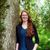QlikView App Dev

Discussion Board for collaboration related to QlikView App Development.

Announcements
QlikWorld 2022, LIVE in Denver CO., May 16-19, 2022. REGISTER NOW TO RECEIVE EARLY BIRD PRICING
cancel
Showing results for
Did you mean:Not applicable

Getting the modal average of a column containing strings

Let's say I have two tables like:

Orders:

Order IDUser ID
ord11
ord22
ord31

Users:

User IDUser Name
1Jordan
2Clive

I have somewhat like the tables above being brought into Qlikview.

Is there a way, in a text object's expression, that I can print the user that has added an order the most amount of times (the Modal Average)? In the example above it would print Jordan, because he has added two orders where Clive has only added 1. This is of course a very simplified example, but it has the basic idea.

1 Solution

Accepted SolutionsMVP

How about something like the attached?

The expression I used in the text box:

=concat({<[User ID]={'=count([Order ID])=\$(=max(aggr(count([Order ID]),[User Name])))'}>}distinct [User Name], ', ')

I used concat() in case there are two (or more users) who have ordered the most amount of times.

3 RepliesMVP

How about something like the attached?

The expression I used in the text box:

=concat({<[User ID]={'=count([Order ID])=\$(=max(aggr(count([Order ID]),[User Name])))'}>}distinct [User Name], ', ')

I used concat() in case there are two (or more users) who have ordered the most amount of times.Not applicable
Author

I'm struggling to figure out how this works - but it does! Thanks a lot.MVP

Okay, I can explain a bit:

Expression:

=concat({<[User ID]={'=count([Order ID])=\$(=max(aggr(count([Order ID]),[User Name])))'}>}distinct [User Name], ', ')

The underlined part counts the number of [Order ID] for each user.

The bold part finds the max number of [Order ID] across all users.

So if the number of [Order ID] for a user is equal to the max number of [Order ID] across all users, we include that [User ID] and use that [User Name] in the concat().

Also, please mark helpful and correct answers so others can find solutions to their problems tooCommunity Browser